## 5.43. balance

Origin

N. Beldiceanu

Constraint

$\mathrm{𝚋𝚊𝚕𝚊𝚗𝚌𝚎}\left(\mathrm{𝙱𝙰𝙻𝙰𝙽𝙲𝙴},\mathrm{𝚅𝙰𝚁𝙸𝙰𝙱𝙻𝙴𝚂}\right)$

Arguments
 $\mathrm{𝙱𝙰𝙻𝙰𝙽𝙲𝙴}$ $\mathrm{𝚍𝚟𝚊𝚛}$ $\mathrm{𝚅𝙰𝚁𝙸𝙰𝙱𝙻𝙴𝚂}$ $\mathrm{𝚌𝚘𝚕𝚕𝚎𝚌𝚝𝚒𝚘𝚗}\left(\mathrm{𝚟𝚊𝚛}-\mathrm{𝚍𝚟𝚊𝚛}\right)$
Restrictions
 $\mathrm{𝙱𝙰𝙻𝙰𝙽𝙲𝙴}\ge 0$ $\mathrm{𝙱𝙰𝙻𝙰𝙽𝙲𝙴}\le \mathrm{𝚖𝚊𝚡}\left(0,|\mathrm{𝚅𝙰𝚁𝙸𝙰𝙱𝙻𝙴𝚂}|-2\right)$ $\mathrm{𝚛𝚎𝚚𝚞𝚒𝚛𝚎𝚍}$$\left(\mathrm{𝚅𝙰𝚁𝙸𝙰𝙱𝙻𝙴𝚂},\mathrm{𝚟𝚊𝚛}\right)$
Purpose

$\mathrm{𝙱𝙰𝙻𝙰𝙽𝙲𝙴}$ is equal to the difference between the number of occurrence of the value that occurs the most and the value that occurs the least within the collection of variables $\mathrm{𝚅𝙰𝚁𝙸𝙰𝙱𝙻𝙴𝚂}$.

Example
 $\left(2,〈3,1,7,1,1〉\right)$ $\left(0,〈3,3,1,1,1,3〉\right)$ $\left(4,〈3,1,1,1,1,1〉\right)$

In the first example, values $1,3$ and 7 are respectively used $3,1$ and 1 times. The corresponding $\mathrm{𝚋𝚊𝚕𝚊𝚗𝚌𝚎}$ constraint holds since its first argument $\mathrm{𝙱𝙰𝙻𝙰𝙽𝙲𝙴}$ is assigned to the difference between the maximum and minimum number of the previous occurrences (i.e., $3-1$). Figure 5.43.1 shows the solution associated with this first example.

##### Figure 5.43.1. Illustration of the first example of the Example slot: five variables ${V}_{1}$, ${V}_{2}$, ${V}_{3}$, ${V}_{4}$, ${V}_{5}$ respectively fixed to values 3, 1, 7, 1 and 1, and the corresponding value of $\mathrm{𝙱𝙰𝙻𝙰𝙽𝙲𝙴}=2$All solutions

Figure 5.43.2 gives all solutions to the following non ground instance of the $\mathrm{𝚋𝚊𝚕𝚊𝚗𝚌𝚎}$ constraint: $\mathrm{𝙱𝙰𝙻𝙰𝙽𝙲𝙴}\in \left[2,3\right]$, ${V}_{1}\in \left[0,5\right]$, ${V}_{2}\in \left[2,6\right]$, ${V}_{3}\in \left[0,1\right]$, ${V}_{4}\in \left[1,2\right]$, $\mathrm{𝚋𝚊𝚕𝚊𝚗𝚌𝚎}$$\left(\mathrm{𝙱𝙰𝙻𝙰𝙽𝙲𝙴},〈{V}_{1},{V}_{2},{V}_{3},{V}_{4}〉\right)$.

##### Figure 5.43.2. All solutions corresponding to the non ground example of the $\mathrm{𝚋𝚊𝚕𝚊𝚗𝚌𝚎}$ constraint of the All solutions slot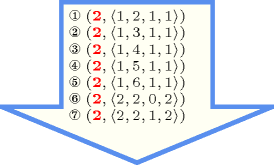Typical
 $\mathrm{𝙱𝙰𝙻𝙰𝙽𝙲𝙴}\le 2+|\mathrm{𝚅𝙰𝚁𝙸𝙰𝙱𝙻𝙴𝚂}|/10$ $|\mathrm{𝚅𝙰𝚁𝙸𝙰𝙱𝙻𝙴𝚂}|>2$
Symmetries
• Items of $\mathrm{𝚅𝙰𝚁𝙸𝙰𝙱𝙻𝙴𝚂}$ are permutable.

• All occurrences of two distinct values of $\mathrm{𝚅𝙰𝚁𝙸𝙰𝙱𝙻𝙴𝚂}.\mathrm{𝚟𝚊𝚛}$ can be swapped; all occurrences of a value of $\mathrm{𝚅𝙰𝚁𝙸𝙰𝙱𝙻𝙴𝚂}.\mathrm{𝚟𝚊𝚛}$ can be renamed to any unused value.

Arg. properties

Functional dependency: $\mathrm{𝙱𝙰𝙻𝙰𝙽𝙲𝙴}$ determined by $\mathrm{𝚅𝙰𝚁𝙸𝙰𝙱𝙻𝙴𝚂}$.

Usage

An application of the $\mathrm{𝚋𝚊𝚕𝚊𝚗𝚌𝚎}$ constraint is to enforce a balanced assignment of values, no matter how many distinct values will be used. In this case one will push down the maximum value of the first argument of the $\mathrm{𝚋𝚊𝚕𝚊𝚗𝚌𝚎}$ constraint.

Remark

If we do not want to use an automaton with an array of counters a possible reformulation of the $\mathrm{𝚋𝚊𝚕𝚊𝚗𝚌𝚎}$ constraint can be achieved in the following way. We use a $\mathrm{𝚜𝚘𝚛𝚝}$ constraint in order to reorder the variables of the collection $\mathrm{𝚅𝙰𝚁𝙸𝙰𝙱𝙻𝙴𝚂}$ and compute the difference between the longest and the smallest sequences of consecutive values.

Counting
 Length ($n$) 2 3 4 5 6 7 8 Solutions 9 64 625 7776 117649 2097152 43046721

Number of solutions for $\mathrm{𝚋𝚊𝚕𝚊𝚗𝚌𝚎}$: domains $0..n$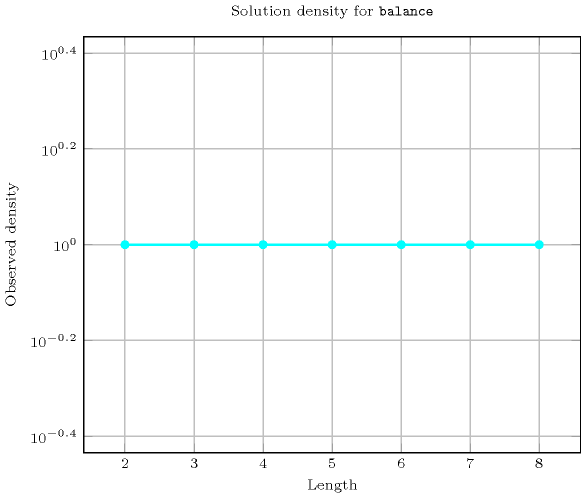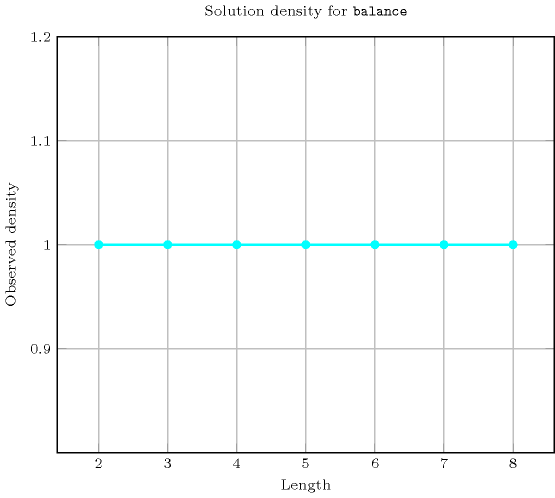Length ($n$)2345678
Total9646257776117649209715243046721
 Parameter value

0928185726861740328682929
1-36360570075600134260024272640
2--8012003003061152015350832
3---1503150952562469600
4----2527056256032
5-----39214112
6------576

Solution count for $\mathrm{𝚋𝚊𝚕𝚊𝚗𝚌𝚎}$: domains $0..n$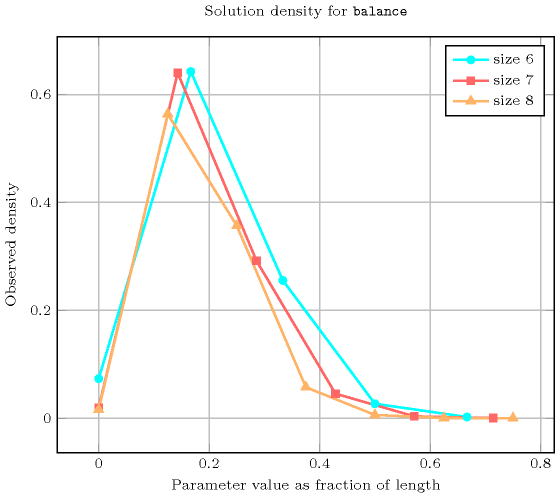generalisation: $\mathrm{𝚋𝚊𝚕𝚊𝚗𝚌𝚎}_\mathrm{𝚒𝚗𝚝𝚎𝚛𝚟𝚊𝚕}$ ($\mathrm{𝚟𝚊𝚛𝚒𝚊𝚋𝚕𝚎}$ replaced by $\mathrm{𝚟𝚊𝚛𝚒𝚊𝚋𝚕𝚎}/\mathrm{𝚌𝚘𝚗𝚜𝚝𝚊𝚗𝚝}$), $\mathrm{𝚋𝚊𝚕𝚊𝚗𝚌𝚎}_\mathrm{𝚖𝚘𝚍𝚞𝚕𝚘}$ ($\mathrm{𝚟𝚊𝚛𝚒𝚊𝚋𝚕𝚎}$ replaced by $\mathrm{𝚟𝚊𝚛𝚒𝚊𝚋𝚕𝚎}\mathrm{mod}\mathrm{𝚌𝚘𝚗𝚜𝚝𝚊𝚗𝚝}$), $\mathrm{𝚋𝚊𝚕𝚊𝚗𝚌𝚎}_\mathrm{𝚙𝚊𝚛𝚝𝚒𝚝𝚒𝚘𝚗}$ ($\mathrm{𝚟𝚊𝚛𝚒𝚊𝚋𝚕𝚎}$ replaced by $\mathrm{𝚟𝚊𝚛𝚒𝚊𝚋𝚕𝚎}\in \mathrm{𝚙𝚊𝚛𝚝𝚒𝚝𝚒𝚘𝚗}$).

related: $\mathrm{𝚋𝚊𝚕𝚊𝚗𝚌𝚎}_\mathrm{𝚌𝚢𝚌𝚕𝚎}$ (balanced assignment versus graph partitionning with balanced cycles), $\mathrm{𝚋𝚊𝚕𝚊𝚗𝚌𝚎}_\mathrm{𝚙𝚊𝚝𝚑}$ (balanced assignment versus graph partitionning with balanced paths), $\mathrm{𝚋𝚊𝚕𝚊𝚗𝚌𝚎}_\mathrm{𝚝𝚛𝚎𝚎}$ (balanced assignment versus graph partitionning with balanced trees), $\mathrm{𝚗𝚟𝚊𝚕𝚞𝚎}$ (no restriction on how balanced an assignment is), $\mathrm{𝚝𝚛𝚎𝚎}_\mathrm{𝚛𝚊𝚗𝚐𝚎}$ (balanced assignment versus balanced tree).

Keywords
Arc input(s)

$\mathrm{𝚅𝙰𝚁𝙸𝙰𝙱𝙻𝙴𝚂}$

Arc generator
$\mathrm{𝐶𝐿𝐼𝑄𝑈𝐸}$$↦\mathrm{𝚌𝚘𝚕𝚕𝚎𝚌𝚝𝚒𝚘𝚗}\left(\mathrm{𝚟𝚊𝚛𝚒𝚊𝚋𝚕𝚎𝚜}\mathtt{1},\mathrm{𝚟𝚊𝚛𝚒𝚊𝚋𝚕𝚎𝚜}\mathtt{2}\right)$

Arc arity
Arc constraint(s)
$\mathrm{𝚟𝚊𝚛𝚒𝚊𝚋𝚕𝚎𝚜}\mathtt{1}.\mathrm{𝚟𝚊𝚛}=\mathrm{𝚟𝚊𝚛𝚒𝚊𝚋𝚕𝚎𝚜}\mathtt{2}.\mathrm{𝚟𝚊𝚛}$
Graph property(ies)
$\mathrm{𝐑𝐀𝐍𝐆𝐄}_\mathrm{𝐍𝐒𝐂𝐂}$$=\mathrm{𝙱𝙰𝙻𝙰𝙽𝙲𝙴}$

Graph class
$\mathrm{𝙴𝚀𝚄𝙸𝚅𝙰𝙻𝙴𝙽𝙲𝙴}$

Graph model

The graph property $\mathrm{𝐑𝐀𝐍𝐆𝐄}_\mathrm{𝐍𝐒𝐂𝐂}$ constraints the difference between the sizes of the largest and smallest strongly connected components.

Parts (A) and (B) of Figure 5.43.3 respectively show the initial and final graph associated with the first example of the Example slot. Since we use the $\mathrm{𝐑𝐀𝐍𝐆𝐄}_\mathrm{𝐍𝐒𝐂𝐂}$ graph property, we show the largest and smallest strongly connected components of the final graph.

##### Figure 5.43.3. Initial and final graph of the $\mathrm{𝚋𝚊𝚕𝚊𝚗𝚌𝚎}$ constraint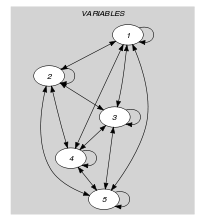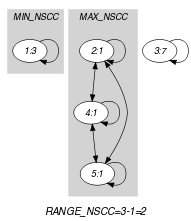(a) (b)
Automaton

Figure 5.43.4 depicts the automaton associated with the $\mathrm{𝚋𝚊𝚕𝚊𝚗𝚌𝚎}$ constraint. To each item of the collection $\mathrm{𝚅𝙰𝚁𝙸𝙰𝙱𝙻𝙴𝚂}$ corresponds a signature variable ${S}_{i}$ that is equal to 1.

##### Figure 5.43.4. Automaton of the $\mathrm{𝚋𝚊𝚕𝚊𝚗𝚌𝚎}$ constraint